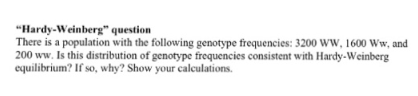# Problem: There is a population with the following genotype frequencies: 3200 WW, 1600 Ww, and 200 ww. Is this distribution of genotype frequencies consistent with Hardy-Weinberg equilibrium? If so, why? Show your calculations.

###### FREE Expert Solution

To know whether the data in the problem is in Hardy-Weinberg Equilibrium, whether there is a significant difference between the observed variables and the expected variables. For this problem we are going to use the Hardy-Weinberg equation expressed as:

p2 + 2pq + q2 = 1###### Problem Details

There is a population with the following genotype frequencies: 3200 WW, 1600 Ww, and 200 ww. Is this distribution of genotype frequencies consistent with Hardy-Weinberg equilibrium? If so, why? Show your calculations.